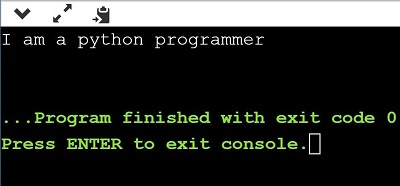# Python Program to Convert List to String

Published January 02, 2021

When you are coding your program in python, we have to convert the list to string many times. Are there any short methods to convert list to string in python? Yes, today in this tutorial you will go through different methods for the python program to convert list to string. Choose one of your best methods and use it in your program to make it more compatible and easy to implement. Here are some examples of conversion of the python program to convert list to string

 Input: ['Python', 'program', 'to', 'convert', 'list', 'to', 'string'] Output: Python program to convert list to string   Input: ['I', 'am', 'learning', 'python', 'programming'] Output: I am learning python programming

Now, let's see various methods of converting the list to string in python.

• Using  .join()
• Using List Comprehension
• Using map()

### Python Program to Convert List to String

#### Method 1- Using iteration & adding the elements.

##### Algorithm-
• First create a function to convert a list to string.

• Now create a string that is empty. Also, get the traverse in the string.

• Return the string and print the output

# Python program to convert a list to string

 # Create a Function to convert  def listToString(p):           # Now create an empty string      s = ""           # To get traverse in the string      for ele in p:          s += ele           # To return string      return s                    #To initialize the list and print the output      p = ['Program', 'in', 'python']  print(listToString(p))

##### Output:

PrograminpythonMethod 2- Using  .join() function

##### Algorithm-
• First create a function to convert a list to string.

• Now create an empty string.

• Return the string and use join() method.

• Initialize input and print the output

# Python program to convert a list to string using .join()

 # Create a Function to convert a list def listToString(p):           # Now create an empty string      s = " "          # return string and use .join() method     return (s.join(p))                    # Initialize and print the output       p = ['python', 'list', 'to', 'string']  print(listToString(p))

##### Output:

python list to stringMethod 3- By using List Comprehension

##### Algorithm-
• Initialize a list in a variable.

• Use list comprehension and .join() method.

• Print the output

# Python program to convert a list to string by using list comprehension

 s = ['I', 'am', 'a', 'python', 'programmer']    # using list comprehension  listToStr = ' '.join([str(elem) for elem in s])    print(listToStr)

##### Output:

I am a python programmerMethod 4-   By using map() function

##### Algorithm-
• Initialize a list.

• Use list comprehension, .join (), .map() method.

• Print the output

# Python program to convert a list to string using map() and list comprehension

 s = ['Python', 'is', 'a', 'programming', 'language']    # Now use list comprehension and .map() function listToStr = ' '.join(map(str, s))    print(listToStr)

 Article Contributed By :625 Views
Projects By category
Categories